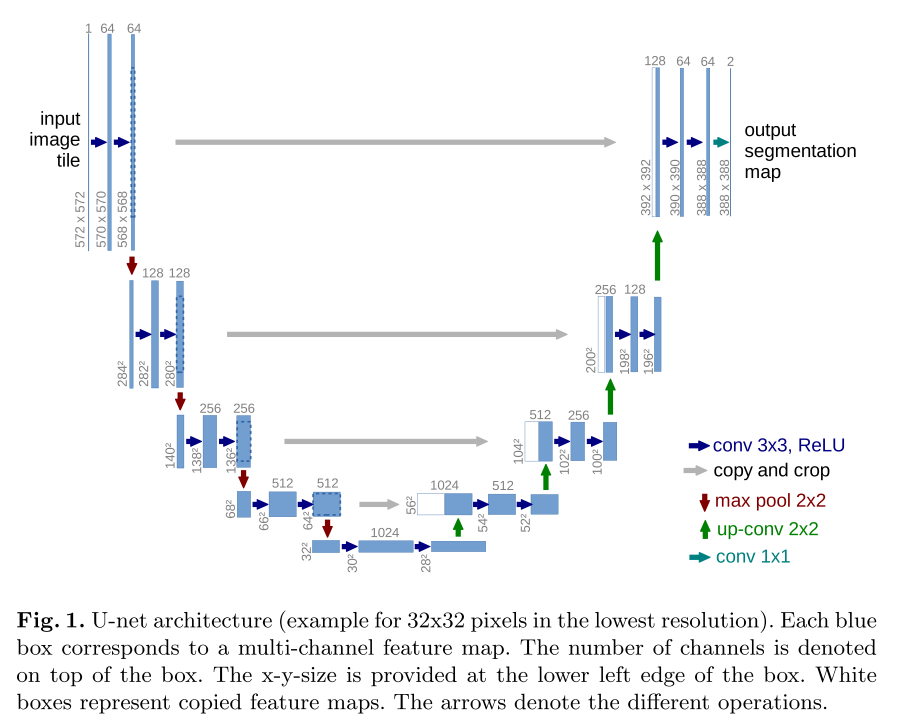## Objective - Implement U-net use Pytorch ## Paper Ronneberger, O., Fischer, P., & Brox, T. (2015). U-net: Convolutional networks for biomedical image segmentation. Lecture Notes in Computer Science (Including Subseries Lecture Notes in Artificial Intelligence and Lecture Notes in Bioinformatics), 9351, 234–241. https://doi.org/10.1007/978-3-319-24574-4_28 ## U-net architectureThe network architecture is illustrated in Figure 1. It consists of a contracting path (left side) and an expansive path (right side). The contracting path follows the typical architecture of a convolutional network. It consists of the repeated application of two 3x3 convolutions (unpadded convolutions), each followed by a rectified linear unit (ReLU) and a 2x2 max pooling operation with stride 2 for downsampling. At each downsampling step we double the number of feature channels. Every step in the expansive path consists of an upsampling of the feature map followed by a 2x2 convolution (“up-convolution”) that halves the number of feature channels, a concatenation with the correspondingly cropped feature map from the contracting path, and two 3x3 convolutions, each fol- lowed by a ReLU. The cropping is necessary due to the loss of border pixels in every convolution. At the final layer a 1x1 convolution is used to map each 64- component feature vector to the desired number of classes. In total the network has 23 convolutional layers. Encoder：左半部分，由两个3x3的卷积层（ReLU）+2x2的max polling层（stride=2）反复组成，每经过一次下采样，通道数翻倍； Decoder：右半部分，由一个2x2的上采样卷积层（ReLU）+Concatenation（crop对应的Encoder层的输出feature map然后与Decoder层的上采样结果相加）+2个3x3的卷积层（ReLU）反复构成； 最后一层通过一个1x1卷积将通道数变成期望的类别数。(Refer: https://zhuanlan.zhihu.com/p/90418337) ## Implementation using Pytorch python # -*- coding: utf-8 -*- """Unet.ipynb Automatically generated by Colaboratory. Original file is located at https://colab.research.google.com/drive/1oLnoOuSmkQjZ998vNvMzhUq_zVq81MPS """ import torch import torch.nn as nn def double_conv(in_c, out_c): conv = nn.Sequential( nn.Conv2d(in_c, out_c, kernel_size=3), nn.ReLU(inplace=True), nn.Conv2d(out_c, out_c, kernel_size=3), nn.ReLU(inplace=True) ) return conv def crop_img(tensor, target_tensor): target_size = target_tensor.size() tensor_size = tensor.size() delta = tensor_size - target_size delta = delta // 2 return tensor[:,:,delta:tensor_size-delta, delta:tensor_size-delta] class UNet(nn.Module): def __init__(self): super(UNet, self).__init__() self.max_pool_2x2 = nn.MaxPool2d(kernel_size=2, stride=2) self.down_con_1 = double_conv(1, 64) self.down_con_2 = double_conv(64, 128) self.down_con_3 = double_conv(128, 256) self.down_con_4 = double_conv(256, 512) self.down_con_5 = double_conv(512, 1024) self.up_trans_1 = nn.ConvTranspose2d(in_channels=1024, out_channels=512, kernel_size=2, stride=2) self.up_cov_1 = double_conv(1024, 512) self.up_trans_2 = nn.ConvTranspose2d(in_channels=512, out_channels=256, kernel_size=2, stride=2) self.up_cov_2 = double_conv(512, 256) self.up_trans_3 = nn.ConvTranspose2d(in_channels=256, out_channels=128, kernel_size=2, stride=2) self.up_cov_3 = double_conv(256, 128) self.up_trans_4 = nn.ConvTranspose2d(in_channels=128, out_channels=64, kernel_size=2, stride=2) self.up_cov_4 = double_conv(128, 64) self.out = nn.Conv2d(in_channels=64, out_channels=2, kernel_size=1) def forward(self, image): # batch size, c, h, w # encode x1 = self.down_con_1(image) # print("x1: ", x1.size()) x2 = self.max_pool_2x2(x1) x3 = self.down_con_2(x2) # x4 = self.max_pool_2x2(x3) x5 = self.down_con_3(x4) # x6 = self.max_pool_2x2(x5) x7 = self.down_con_4(x6) # x8 = self.max_pool_2x2(x7) x9 = self.down_con_5(x8) print("x9: ", x9.size()) # decoder x = self.up_trans_1(x9) y = crop_img(x7, x) x = self.up_cov_1(torch.cat([x, y], 1)) x = self.up_trans_2(x) y = crop_img(x5, x) x = self.up_cov_2(torch.cat([x, y], 1)) x = self.up_trans_3(x) y = crop_img(x3, x) x = self.up_cov_3(torch.cat([x, y], 1)) x = self.up_trans_4(x) y = crop_img(x1, x) x = self.up_cov_4(torch.cat([x, y], 1)) y = self.out(x) # print(x.size()) print("output:", y.size()) if __name__ == "__main__": image = torch.rand((1, 1, 572, 572)) print("Input:", image.size()) model = UNet() print(model(image))  ## References - [Implementing original U-Net from scratch using PyTorch](https://www.youtube.com/watch?v=u1loyDCoGbE) - [paper阅读笔记 UNet](https://zhuanlan.zhihu.com/p/90418337)